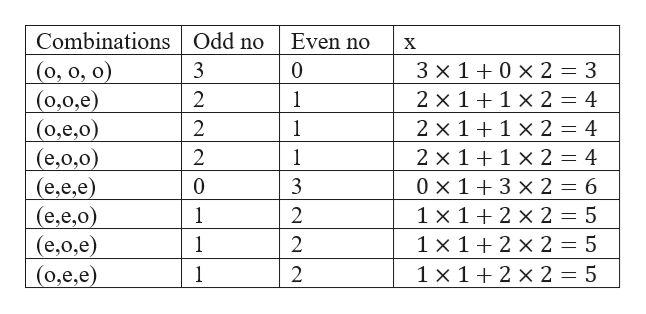# Assume that the box contains balls numbered from 1 through 26, and that 3 are selected. A random variable XX is defined as 1 times the number of odd balls selected, plus 2 times the number of even.How many different values are possible for the random variable XX?   Fill in the table below to complete the probability density function. Be certain to list the values of XX in ascending order. Value of XProbability         N

Question
372 views

Assume that the box contains balls numbered from 1 through 26, and that 3 are selected. A random variable XX is defined as 1 times the number of odd balls selected, plus 2 times the number of even.

How many different values are possible for the random variable XX?

Fill in the table below to complete the probability density function. Be certain to list the values of XX in ascending order.

 Value of X Probability

N

check_circle

Step 1

As per the question, the number can be either odd or even and 3 are selected

So total possible combinations are 23= 8

Out of 26, there are 13 odd and ...help_outlineImage TranscriptioncloseOdd no Combinations Even no х (o, o, o) (0,0,e) (0,е,0) (e,o,0) (e,e,e) (e,e,0) (е,0,е) (0,e,e) 3 0 3 x 10 x 2 = 3 2 1 2 x 11 x 2 = 4 2 1 2 x 1 1 x 2 = 4 2 1 2 x 11 x 2 = 4 0 x 13 x 2 = 6 1 x 12 x 2 = 5 1 x 1 2 x 2 = 5 0 3 - 1 2 1 2 1 1 x 12 x 2 = 5 fullscreen

### Want to see the full answer?

See Solution

#### Want to see this answer and more?

Solutions are written by subject experts who are available 24/7. Questions are typically answered within 1 hour.*

See Solution
*Response times may vary by subject and question.
Tagged in

### Probability